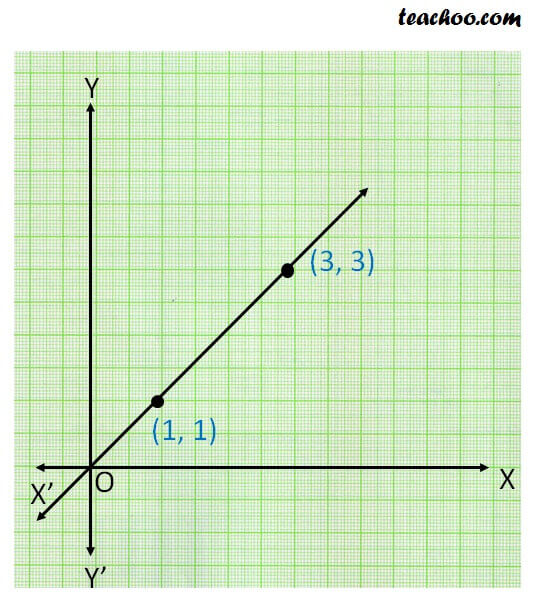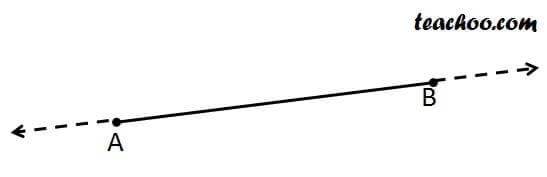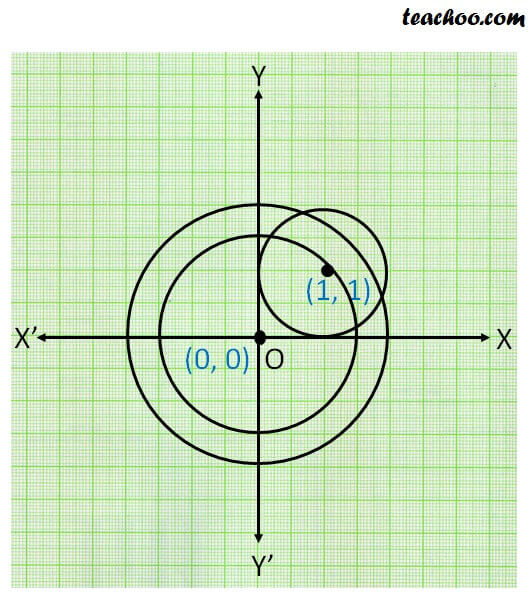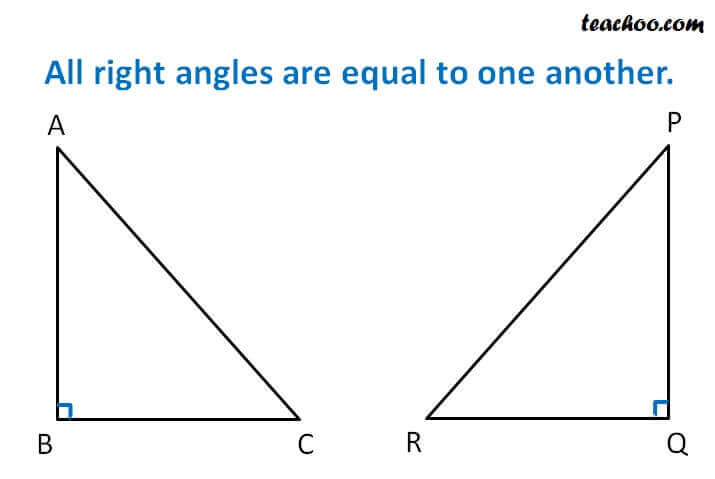Postulates

Chapter 5 Class 9 Introduction to Euclid's Geometry
Concept wise

## Postulate 1:

A straight line may be drawn from any one point to any other point.

The postulate says that a line passes through two point.

But, it does not say that only one line passes through 2 distinct points .

So, we make an axiom of it -  Axiom 5.1## Postulate 2:

Terminated line means line segment can be extended from either

side to form a lines.Here, Line AB can be extended from both sides

## Postulate 3:

A circle can be drawn with any center and any radius

Eg: Circle of radius 1.5 cm & centre (0, 0)

Circle of radius 2 cm & centre (0, 0)

Circle of radius 1 cm & centre (1, 1)## Postulate 4:

All right angles are equal to one another .Here, ∠ B = ∠ Q = 90°

## Postulate 5:

If a straight line falling on two straight lines makes the interior

angles on the same side of it taken together less than two right angles, then the

two straight lines, if produced indefinitely, meet on that side on which the sum of

angles is less than two right angles

We discuss more about it in

Learn in your speed, with individual attention - Teachoo Maths 1-on-1 Class